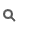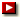# 機率與統計(Probabiltiy and Statistics)

 Instructor: Prof. Hsueh-Ming Hang (杭學鳴教授) Office: ED609 x 31861 Email: hmhang@mail.nctu.edu.tw Lecture: 1B, 5EF, ED116Office hours:Teaching Assistant:Course Description:
This course gives an introduction to basic concepts and computations of elementary probability theory. We cover the following topics: axioms of probability, joint and conditional probability, random variables, functions of one and two random variables, expected value and moments, central limit theorem, laws of large numbers, and basic statistical analysis tools. They constitute the fundamental knowledge of electrical engineering and computer science disciplines, particularly in the areas of communications, networking and signal processing.公告(Announcement):Reference Web Site:Text book:
D. P. Bertsekas and J. N. Tsitskilis, Introduction to Probability, Athena Scientific, 2nd edition, 2008Grading:
Homework: 20%
Quiz 1: 10%
Quiz 2: 10%
Midterm Examine: 30% (2 hours, closed book)
Final Examine: 30% (2 hours, closed book)Backgorund:
Calculus, Linear algebraContents:Homework:Supplements: